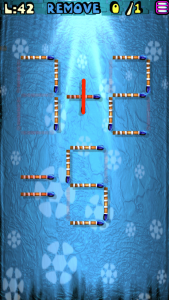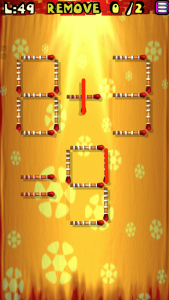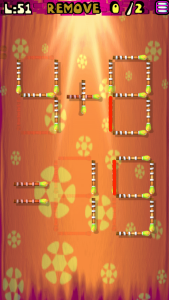# Move the Matches Puzzles Episode 2 Level 41-60 Walkthrough

Walkthrough for all the solutions and answers to the game Move the Matches Puzzles by K-Lab. Get the app on the Google Play Store to play along if you do not already have it.  This is for episode 2, levels 41 through 60.

### Level 41Instructions: Add 4 matches to correct the equation

Solution: 4×7=28

### Level 42Instructions: Remove 1 match to correct the equation

Solution: 7-2=5

### Level 43Instructions: Move 2 matches to correct the equation

Solution: 6×3=18

### Level 44Instructions: Move 2 matches to get 3 triangles

### Level 45Instructions: Add 2 matches to correct the equation

### Level 46Instructions: Move 2 matches to get 2 triangles

### Level 47Instructions: Move 4 matches to get 2 squares

### Level 48Instructions: Add 3 matches to correct the equation

Solution: 7×8=56

### Level 49Instructions: Remove 2 matches to correct the equation

Solution: 8-3=5

### Level 50Instructions: Add 2 matches to get 6 squares

### Level 51Instructions: Remove 2 matches to correct the equation

Solution: 4+9=13

### Level 52Instructions: Move 2 matches to get 4 squares

### Level 53Instructions: Remove 1 match to correct the equation

Solution: 3×2=6

### Level 54Instructions: Move 2 matches to correct the equation

Solution: 7+6=13

### Level 55Instructions: Remove 2 matches to correct the equation

Solution: 7-6=1

### Level 56Instructions: Remove 2 matches to get 6 squares, all equal

### Level 57Instructions: Add 2 matches to correct the equation

Solution: 7×9=63

### Level 58Instructions: Move 3 matches to get 4 squares

### Level 59Instructions: Move 4 matches to get 4 squares

### Level 60Instructions: Remove 4 matches to get 8 triangles, all equal